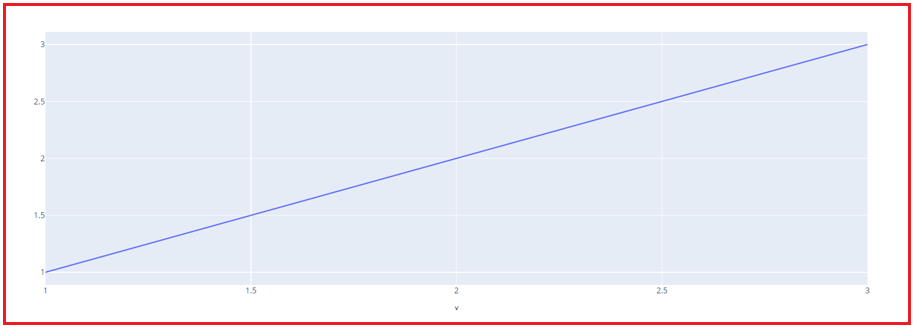# Introduction to Plotly

## Introduction to Python Plotly for Data Science

In this article, I am going to give you a brief introduction to Python Plotly for Data Science. At the end of this article, you will understand what is Plotly, how to use Plotly, and some basic concepts of Plotly with examples.

##### What is Plotly?

The Plotly Python library is an interactive, open-source plotting toolkit that supports over 40 different chart types for statistical, financial, geographic, scientific, and 3-dimensional applications.

Plotly is a Python library that allows users to create beautiful interactive web-based visualizations that can be displayed in Jupyter notebooks, saved to standalone HTML files, or served as part of pure Python-built web applications using Dash. It is built on top of the Plotly JavaScript library (plotly.js). To distinguish it from the JavaScript library, the Plotly Python library is commonly referred to as “plotly.py.”

Recently Plotly has gained a lot of popularity. So, people are preferring Plotly over other libraries? Here’s the answer.

1. Hover tool capabilities in Plotly allow us to identify outliers or anomalies in massive numbers of knowledge points.
2. It has an aesthetically appealing design that appeals to a wide spectrum of people.
3. It allows us to customize our graphs in an infinite number of ways, making our plots more interesting and understandable to others.

To start working on Plotly, you need to install it first. This is how you can do it –

pip install plotly

##### Python Plotly Basics

Let’s have a look at the structure of the Plotly package and what all it has to offer. Plotly is divided into three basic parts. They are as follows:

1. plotly.plotly
2. plotly.graph.objects
3. plotly.tools

1. Plotly communicates with the local machine through plotly.plotly. It has features that rely on Plotly’s server to respond.

2. The plotly.graph.objects module contains the objects that are responsible for constructing plots (figure, layout, data, and plot definitions such as scatter plots and line charts).

3. The plotly.tools module offers a variety of tools within the sort of functions which will be wont to improve your Plotly experience.

Let’s have a look at one example of how to visualize data using Plotly. To create easy to style and an entire figure at once, we will use plotly.express

```# Importing necessary libraries
# Using plotly.express for creating figures at once
import plotly.express as px

# Creating the Figure instance
# Creating a line plot for x and y data points
figure = px.line(x=[1,2,3], y=[1,2,3])

# displaying the figure instance
figure.show()
```
###### Output:In the next article, I am going to discuss Line Charts in Python using Plotly for Data Science with Examples. Here, in this article, I try to give an overview of Introduction to Plotly for Data Science. I hope you enjoy this Plotly for Data Science article.